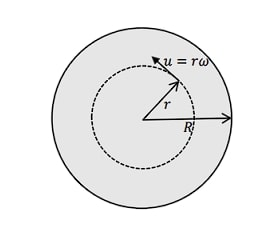Please help me in solving this. I am unable to understand how to approach this question. Please explain this briefly to me along with some hints on how to approach the three parts.

1 лайк

This problem was in the Taiwan team training camp material. I translated it into English.

Anyone?

Yes, this problem is quite hard to grasp, but as far as I understood, the first part of this problem simply wants us to calculate the surface charge distribution on conductive cylinder under the external electric field perpendicular to cylinder’s axis. If you remember, the distribution obeys the \sigma(\theta)=\sigma_0\cos\theta law, and in order to find \sigma_0, you should plug in a condition that the electric field inside the cylinder is zero.

As you solve the first part, for the second one you immediately come to an idea of calculating torque through \vec\tau =\vec p\times\vec E.

The net torque found in the second part equals the torque caused by relative motion of inner layers (R<r<R+h) of the viscous fluid.

2 лайка

Oh, thanks… let me try through this way